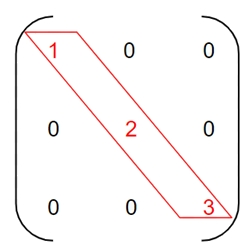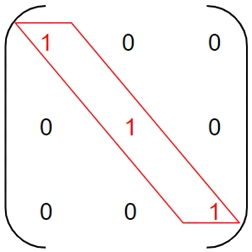# Program to check diagonal matrix and scalar matrix in C++

Given a matrix M[r][c], ‘r’ denotes number of rows and ‘c’ denotes number of columns such that r = c forming a square matrix. We have to find whether the given square matrix is diagonal and scalar matrix or not, if it is diagonal and scalar matrix then print yes in the result.

## Diagonal matrix

A square matrix m[][] will be diagonal matrix if and only if the elements of the except the main diagonal are zero.

Like in the given figure below −Here, the elements in the red are main diagonal which are non-zero rest elements except the main diagonal are zero making it a Diagonal matrix.

## Example

Input: m = { {7, 0, 0},
{0, 8, 0},
{0, 0, 9}}
Output: yes
Input: m = {  {1, 2, 3},
{0, 4, 0},
{0, 0, 5}
}
Output: no

## Algorithm

Start
Step 1 -> define macro of size 4
Step 2 -> declare function to check if matrix is diagonal or not
bool ifdiagonal(int arr[size][size])
Loop For int i = 0 and i < size and i++
Loop for int j = 0 and j < size and j++
IF ((i != j) & (arr[i][j] != 0))
return false
End
End
End
return true
step 3 -> In main()
Declare and set int arr[size][size] = { { 1, 0, 0, 0 },
{ 0, 1, 0, 0 },
{ 0, 0, 1, 0 },
{ 0, 0, 0, 1 }
};
IF (ifdiagonal(arr))
Print its a diagonal matrix
End
Else
Print its not a diagonal matrix
End
Stop

## Diagonal Matrix

### Example

#include <bits/stdc++.h>
#define size 4
using namespace std;
// check if matrix is diagonal matrix or not.
bool ifdiagonal(int arr[size][size]){
for (int i = 0; i < size; i++)
for (int j = 0; j < size; j++)

if ((i != j) && (arr[i][j] != 0))
return false;
return true;
}
int main(){
int arr[size][size] = { { 1, 0, 0, 0 },
{ 0, 1, 0, 0 },
{ 0, 0, 1, 0 },
{ 0, 0, 0, 1 }
};
if (ifdiagonal(arr))
cout << "its a diagonal matrix" << endl;
else
cout << "its not a diagonal matrix" << endl;
return 0;
}

### Output

its a diagonal matrix

## SCALAR MATRIX

A square matrix m[][] is Scalar Matrix if the elements in the main diagonal are equal and the rest of the elements are zero.

Like in the given example below −Here, the elements in the red are the diagonal elements which are same and rest elements are zero making it a Scalar Matrix

## Example

Input: m = { {2, 0, 0},
{0, 2, 0},
{0, 0, 2} }
Output: yes
Input: m = { {3, 0, 0},
{0, 2, 0},
{0, 0, 3} }
Output: no

## Algorithm

Start
Step 1 -> Declare macro as #define size 4
Step 2 -> declare function to check matrix is scalar matrix or not.
bool scalar(int arr[size][size])
Loop For int i = 0 and i < size and i++
Loop For int j = 0 and j < size and j++
IF ((i != j) && (arr[i][j] != 0))
return false
End
End
End
Loop for int i = 0 and i < size – 1 and i++
If (arr[i][i] != arr[i + 1][i + 1])
return false
End
End
Return true
Step 3 -> In main()
Declare array as int arr[size][size] = { { 2, 0, 0, 0 },
{ 0, 2, 0, 0 },
{ 0, 0, 2, 0 },
{ 0, 0, 0, 2 }
}
IF(scalar(arr))
Print its a scalar matrix
Else
Print its not a scalar matrix
Stop

## Example

#include <bits/stdc++.h>
#define size 4
using namespace std;
// check matrix is scalar matrix or not.
bool scalar(int arr[size][size]){
for (int i = 0; i < size; i++)
for (int j = 0; j < size; j++)
if ((i != j) && (arr[i][j] != 0))
return false;
for (int i = 0; i < size - 1; i++)
if (arr[i][i] != arr[i + 1][i + 1])
return false;
return true;
}
int main(){
int arr[size][size] = { { 2, 0, 0, 0 },
{ 0, 2, 0, 0 },
{ 0, 0, 2, 0 },
{ 0, 0, 0, 2 } };
if (scalar(arr))
cout << "its a scalar matrix" << endl;
else
cout << "its not a scalar matrix" << endl;
return 0;
}

## Output

its a scalar matrix